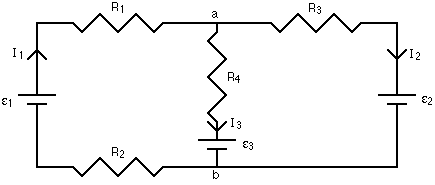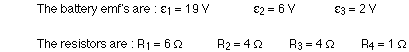## Circuit Lab B/C

mjcox2000
MemberPosts: 121
Joined: May 9th, 2014, 3:34 am
State: VA
Has thanked: 0
Been thanked: 0

### Re: Circuit Lab B/C

UTF-8 U+6211 U+662F wrote:
mjcox2000 wrote:
your third equation should have $I-\frac{V}{R_p}$, not $I-VR_p$, and that error messed up almost everything else you have. With that fix, you would have gotten part a right, and I haven't evaluated part b, but the way you approach it seems reasonable. Wolfram Alpha or Mathematica might help with the more involved integrals.
Agh! Well that sucks.
a)

$Q=CV$

$I_C=C \cdot \frac{dV}{dt}$

$I-\frac{V}{R_p} = C \cdot \frac{dV}{dt}$

$dt = C \cdot \frac{dV}{I-\frac{V}{R_p}}$

$t + C_{int} = -CR_p\ln(I-\frac{V}{R_p})$ (the constant of integration also being a C makes this slightly confusing)

$t = -CR_p\ln(I-\frac{V}{R_p}) + k$ (so I changed it to a k)

$t = -CR_p\ln(I-\frac{V}{R_p}) + CR_p\ln I$ (but plugging in (0,0) gives us a value for k)

$\boxed{t_f = -CR_p\ln(I-\frac{V_f}{R_p}) + CR_p\ln I}$

b) I'm going to assume you mean energy for the next problem?

$P = I^2R_s + \frac{V^2}{R_p}$

$E = I^2R_s \cdot (-CR_p\ln(I-\frac{V_f}{R_p}) + CR_p\ln I) + \int^{t_f}_0\frac{V^2}{R_p}dt$

Now, let's solve for V in terms of t...

$t = -CR_p\ln(I-\frac{V}{R_p}) + CR_p\ln I$

$\frac{CR_p\ln I - t}{CR_p} = \ln(I-\frac{V}{R_p})$

$\ln I - \frac{t}{CR_p} = \ln(I-\frac{V}{R_p})$

$Ie^{-\frac{t}{CR_p}} = I - \frac{V}{R_p}$

$V = IR_p(1-e^{-\frac{t}{CR_p}})$

$E = CI^2R_pR_s\ln\frac{I}{I-\frac{V_f}{R_p}} + \int^{t_f}_0\frac{(IR_p(1-e^{-\frac{t}{CR_p}}))^2}{R_p}dt$

$E = CI^2R_pR_s\ln\frac{I}{I-\frac{V_f}{R_p}} + I^2R_p\int^{t_f}_0(1-e^{-\frac{t}{CR_p}})^2dt$

$E = CI^2R_pR_s\ln\frac{I}{I-\frac{V_f}{R_p}} + I^2R_p\left[t+\frac{e^{-2\frac{t}{CR_p}}-4e^{{-\frac{t}{CR_p}}}}{-\frac{2}{CR_p}}\right]^{t_f}_0$

$\boxed{E = CI^2R_pR_s\ln\frac{I}{I-\frac{V_f}{R_p}} + I^2R_p\left(-CR_p\ln(I-\frac{V_f}{R_p}) + CR_p\ln I+\frac{\frac{(I-\frac{V_f}{R_p})^2}{I^2}-4\frac{I-\frac{V_f}{R_p}}{I}}{-\frac{2}{CR_p}}-\frac{3CR_p}{2}\right)}$

For part c:

$\boxed{0 = \frac{d}{dV}\left[CI^2R_pR_s\ln\frac{I}{I-\frac{V_f}{R_p}} + I^2R_p\left(-CR_p\ln(I-\frac{V_f}{R_p}) + CR_p\ln I+\frac{\frac{(I-\frac{V_f}{R_p})^2}{I^2}-4\frac{I-\frac{V_f}{R_p}}{I}}{-\frac{2}{CR_p}}-\frac{3CR_p}{2}\right)\right]}$

Although you'd need to test all of the roots and see which one yields the lowest value
That's right!
Instead of integrating for $V_{capacitor}(t)$, you can eliminate $R_s$ from the circuit since it impacts just the power loss and not the charging time. Then, $I$ is in parallel with $R_p$, and you can use Norton-Thevenin equivalence to see that the capacitor is simply charged by a voltage source $IR_p$ through resistance $R_p$, so you can apply a stock formula rather than integrating.

Your part b formula is right, but it can be algebraically simplified to $-\frac12 c \bigg[V_f (2 I R_p + V_f) + 2 I^2 R_p (R_p + R_s) ln\bigg(1 - \frac{V_f}{iR_p}\bigg)\bigg].$

I haven't proved it, but intuition tells me there can only be one point at which the derivative of part b is 0. If current is under $V_f/R_p$, infinite energy is wasted because the target voltage is never reached. With current slightly above that threshold, it takes a very long time to charge, so a lot of energy is wasted in the series resistor. As current increases, time decreases, which should monotonically decrease the energy wasted in the series resistor. However, when current is very high, the parallel resistor wastes a lot of energy because it shorts around the capacitor. If I'm not mistaken, the limiting energy waste for small and large currents mean that there should be just one local minimum. (Plots I've done seem to confirm that.)
MIT ‘23
TJHSST ‘19
Longfellow MS

See my user page for nationals medals and event supervising experience.

UTF-8 U+6211 U+662F
Exalted MemberPosts: 1523
Joined: January 18th, 2015, 7:42 am
Division: C
State: PA
Has thanked: 1 time
Been thanked: 1 time

### Re: Circuit Lab B/C

Explain how you could solve for the resistance between two ends of a bridge circuit using Kirchoff's rules.

wec01
MemberPosts: 220
Joined: February 22nd, 2019, 4:02 pm
State: VA
Has thanked: 0
Been thanked: 0

### Re: Circuit Lab B/C

UTF-8 U+6211 U+662F wrote:Explain how you could solve for the resistance between two ends of a bridge circuit using Kirchoff's rules.
I believe you could just use Kirchoff's loop rule to set up three equations involving different currents then use algebra to determine the total current. From there you could just use Ohm's law to solve for the total resistance.
2019 Division C Nationals Medals:
4th place Fossils
5th place Sounds of Music
2nd place Thermodynamics

UTF-8 U+6211 U+662F
Exalted MemberPosts: 1523
Joined: January 18th, 2015, 7:42 am
Division: C
State: PA
Has thanked: 1 time
Been thanked: 1 time

### Re: Circuit Lab B/C

wec01 wrote:
UTF-8 U+6211 U+662F wrote:Explain how you could solve for the resistance between two ends of a bridge circuit using Kirchoff's rules.
I believe you could just use Kirchoff's loop rule to set up three equations involving different currents then use algebra to determine the total current. From there you could just use Ohm's law to solve for the total resistance.
Yep, your turn. Also mention source injection, as applying Kirchoff's laws is rather difficult without it.

wec01
MemberPosts: 220
Joined: February 22nd, 2019, 4:02 pm
State: VA
Has thanked: 0
Been thanked: 0

### Re: Circuit Lab B/CCalculate the values of I1, I2, and I3 given:2019 Division C Nationals Medals:
4th place Fossils
5th place Sounds of Music
2nd place Thermodynamics

Cathy-TJ
MemberPosts: 28
Joined: January 20th, 2019, 7:13 pm
Division: C
State: VA
Has thanked: 0
Been thanked: 0

### Re: Circuit Lab B/C

wec01 wrote:Calculate the values of I1, I2, and I3 given:I1 = 1.5 A
I2 = -0.5 A
I3 = 2 A
Nationals Placings:
2019 Circuit Lab - 1
2018 Disease Detectives - 1
2015 Picture This - 6

2019 Events:
Chemistry Lab, Circuit Lab, Disease Detectives

Competitors today, teammates tomorrow

mjcox2000
MemberPosts: 121
Joined: May 9th, 2014, 3:34 am
State: VA
Has thanked: 0
Been thanked: 0

### Re: Circuit Lab B/C

Edit: Cathy-TJ beat me to an answer.
MIT ‘23
TJHSST ‘19
Longfellow MS

See my user page for nationals medals and event supervising experience.

wec01
MemberPosts: 220
Joined: February 22nd, 2019, 4:02 pm
State: VA
Has thanked: 0
Been thanked: 0

### Re: Circuit Lab B/C

Cathy-TJ wrote:
wec01 wrote:Calculate the values of I1, I2, and I3 given:I1 = 1.5 A
I2 = -0.5 A
I3 = 2 A
2019 Division C Nationals Medals:
4th place Fossils
5th place Sounds of Music
2nd place Thermodynamics

Cathy-TJ
MemberPosts: 28
Joined: January 20th, 2019, 7:13 pm
Division: C
State: VA
Has thanked: 0
Been thanked: 0

### Re: Circuit Lab B/C

Calculate the resistance between terminals A and B in the infinite chain of resistors where all resistors are 1 ohm.
Nationals Placings:
2019 Circuit Lab - 1
2018 Disease Detectives - 1
2015 Picture This - 6

2019 Events:
Chemistry Lab, Circuit Lab, Disease Detectives

Competitors today, teammates tomorrow

mjcox2000
MemberPosts: 121
Joined: May 9th, 2014, 3:34 am
State: VA
Has thanked: 0
Been thanked: 0

### Re: Circuit Lab B/C

Cathy-TJ wrote:Calculate the resistance between terminals A and B in the infinite chain of resistors where all resistors are 1 ohm.
Since the network repeats, if its resistance is R, it consists of a 1-ohm resistor in series with a unit consisting of a parallel 1-ohm and R-ohm resistor. Solving for R, we see that $R=\frac{1+\sqrt5}2\Omega$, or assuming sufficient sig figs, about $1.618\Omega$.